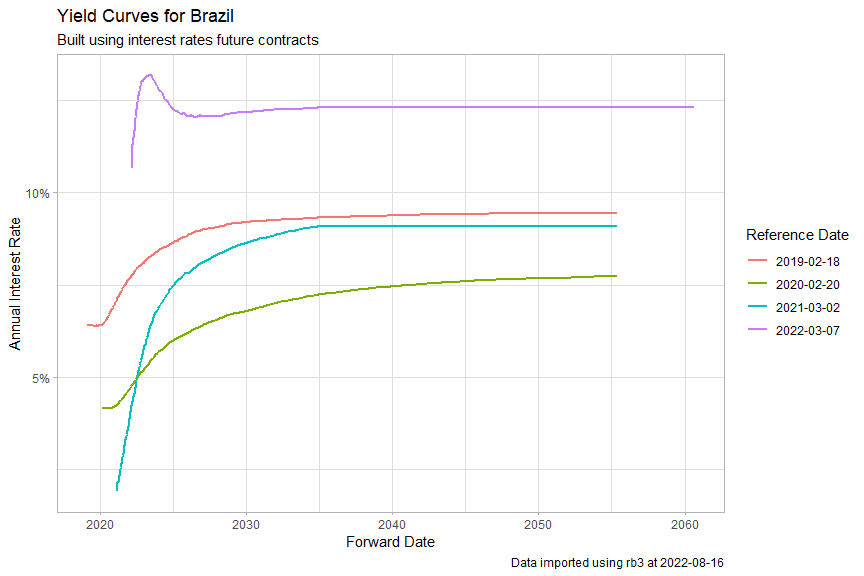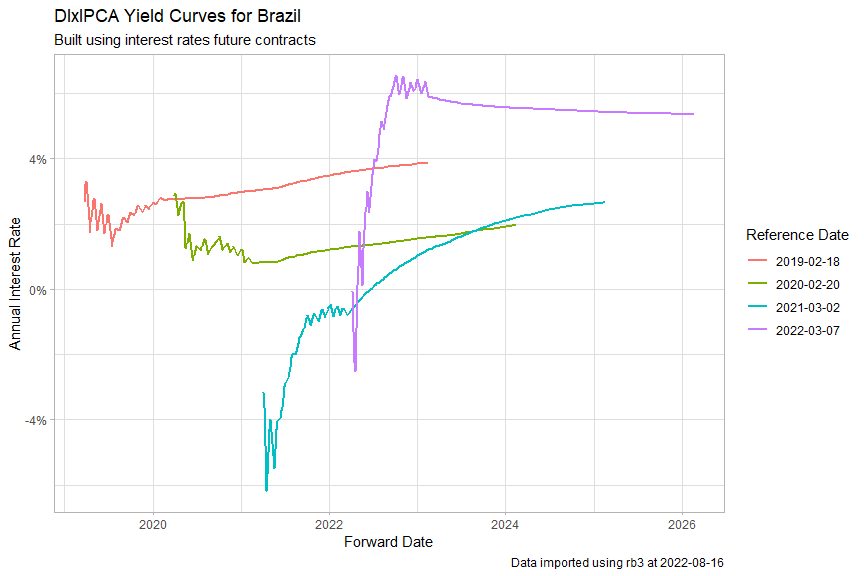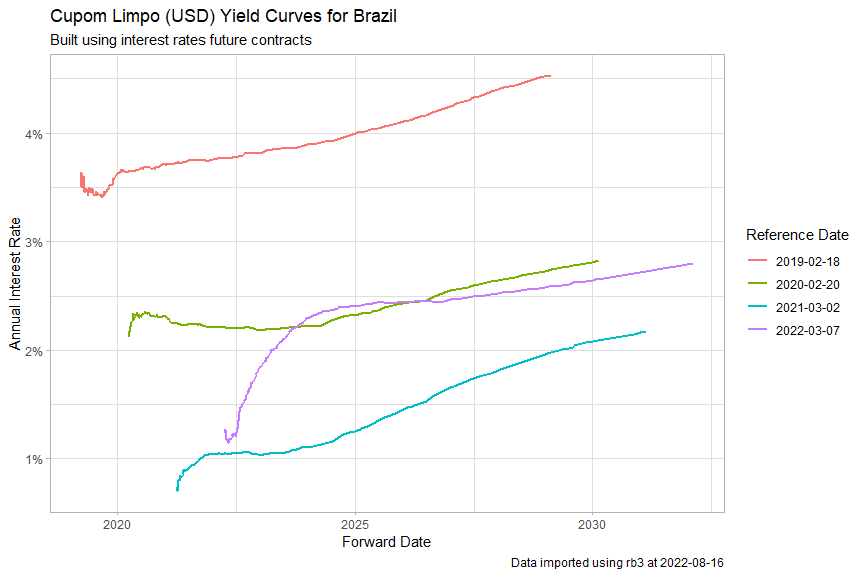# Fetching Historical Yield Curves from B3

Data for the yield curve is available in B3’s website. The data is built using interest rate futures. See this pdf for more details about the source of the yield curves.

library(rb3)
library(ggplot2)
library(stringr)
library(dplyr)

## DI X Pre Curve

df_yc <- yc_mget(
first_date = Sys.Date() - 255 * 5,
last_date = Sys.Date(),
by = 255
)

p <- ggplot(
df_yc,
aes(
x = forward_date,
y = r_252,
group = refdate,
color = factor(refdate)
)
) +
geom_line(size = 1) +
labs(
title = "Yield Curves for Brazil",
subtitle = "Built using interest rates future contracts",
caption = str_glue("Data imported using rb3 at {Sys.Date()}"),
x = "Forward Date",
y = "Annual Interest Rate",
color = "Reference Date"
) +
theme_light() +
scale_y_continuous(labels = scales::percent)

print(p)## DI x IPCA Curve

df_yc <- yc_ipca_mget(
first_date = Sys.Date() - 255 * 5,
last_date = Sys.Date(),
by = 255
)

p <- ggplot(
df_yc |> filter(biz_days > 21, biz_days < 1008),
aes(
x = forward_date,
y = r_252,
group = refdate,
color = factor(refdate)
)
) +
geom_line(size = 1) +
labs(
title = "DIxIPCA Yield Curves for Brazil",
subtitle = "Built using interest rates future contracts",
caption = str_glue("Data imported using rb3 at {Sys.Date()}"),
x = "Forward Date",
y = "Annual Interest Rate",
color = "Reference Date"
) +
theme_light() +
scale_y_continuous(labels = scales::percent)

print(p)## Cupom Limpo (USD)

df_yc <- yc_usd_mget(
first_date = Sys.Date() - 255 * 5,
last_date = Sys.Date(),
by = 255
)

p <- ggplot(
df_yc |> filter(biz_days > 21, biz_days < 2520),
aes(
x = forward_date,
y = r_360,
group = refdate,
color = factor(refdate)
)
) +
geom_line(size = 1) +
labs(
title = "Cupom Limpo (USD) Yield Curves for Brazil",
subtitle = "Built using interest rates future contracts",
caption = str_glue("Data imported using rb3 at {Sys.Date()}"),
x = "Forward Date",
y = "Annual Interest Rate",
color = "Reference Date"
) +
theme_light() +
scale_y_continuous(labels = scales::percent)

print(p)